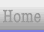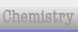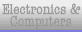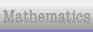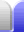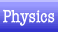# The Most Famous Equation in Physics

E = mc2 is the most famous equation in physics; almost everyone with a reasonable education has heard of it. It describes the equivalence of energy and matter. Energy can be converted into matter, and matter can be converted into energy. This happens in nuclear reactions.

E = mc2
energy = mass x (speed of light)2

The speed of light is a constant, so its square is also going to be a constant – and a rather big number – so a large amount of energy is equivalent to a small amount of mass. The normal units used:

joules = kilograms x (metres per second)2
J = kg·m2·s-2
J = kg·m2/s2

Note that the kilogram is the only base unit which has a prefix. There are historical reasons for that.

If we want to convert energy to mass we rearrange the equation.

m = E / c2
mass = energy / (speed of light)2

### How much energy does 1 kg equate to?

Let's use this equation to work out how much energy is in 1 kg.

E = mc2
E = 1 x 300,000,0002
= 9 x 1016 J
= 90 petajoules

To put that into some sort of perspective, 90 petajoules represents about 2.5 billion litres of petrol, or 21.5 megatonnes of TNT.

90 petajoules is just over the amount of electricity New Zealand produced from hydroelectric power stations in 2014.

### How much mass converted to energy would it take to boil the world's ocean?

The average ocean surface temperature is 17°C, and since it's at sea level (!) it boils at 100°C. Water takes about 4,180 J to heat 1 kilogram up by 1°C (the specific heat) and 2,260,000 J to turn 1 kg of water at 100°C into steam at 100°C (the latent heat of vaporisation). The average ocean depth is around 3,000 meters.

Earth's circumference (through the poles) is 40,000 km. That's because the metre was originally defined in 1793 as being one ten millionth the distance from the north pole to the equator (through Paris). The whole circumference is thus 40 million metres.

circumference = 2π x radius
r = c / 2π
Earth's radius = 40,000,000 / 2π
= 6,370,000 m

volume of a sphere = 4/3πr3
volume of ocean = 4/3π(6.37 x 106)3 - 4/3π(6.367 x 106)3
= 4/3π( (6.37 x 106)3 - (6.367 x 106)3 )
= 1.53 x 1018 m3
= 1.53 x 1021 kg

energy to heat to 100°C = 4,180 x (100 - 17) x 1.53 x 1021
= 5.31 x 1026 J
mass equivalent = 5.31 x 1026 / (3 x 108)2
= 5.9 x 109 kg
= 5.9 billion kilograms

energy to evaporate = 2,260,000 x 1.53 x 1021
= 3.46 x 1027 J
mass equivalent = 3.46 x 1027 / (3 x 108)2
= 3.84 x 1010 kg
= 38.4 billion kilograms

So a total of 44.3 billion kilograms of mass converted to energy would be needed to heat and boil off the world's oceans. This is a lot of energy, and one reason why Earth is a nice place to live – all that water provides a huge stablising effect.

### How much mass does the Sun convert into energy each second?

In the Sun four hydrogen atoms are converted (in several steps) into a single helium atom, but the helium atom does not have the same mass as the four hydrogen atoms it was formed from. Most of that lost mass has been converted to energy (a few weird particles are emitted as well).

We could just look up the solar luminance and plug that into E = mc2, but let's back up a step or two and work it out from how much energy Earth receives each second. Wikipedia:

The Earth receives 174,000 terawatts (TW) of incoming solar radiation (insolation) at the upper atmosphere. Approximately 30% is reflected back to space while the rest is absorbed by clouds, oceans and land masses.

If we divide 174 petawatts, or 1.74 x 1017 W, by the cross-sectional area of Earth, we'll get the power per square meter at one astronomical unit (1 AU, Earth's average orbit radius) from the Sun.

area of a circle = πr2
Earth's cross-section area = π x 6,370,0002
= 1.27 x 1014 m2

solar irradiance = power / area
solar irradiance at 1 AU = 1.74 x 1017 W / 1.27 x 1014 m2
= 1.37 kW/m2

That's the amount of solar radiation at a range of 1 AU. We just need to multiply this by the surface area of a sphere with radius of 1 AU to get the power output of the Sun, and hence the energy it delivers each second (because 1 W = 1 J/s). 1 AU is about 150 million kilometres (150 billion metres).

surface area of sphere = 4πr2
= 4π x 150,000,000,0002
= 2.83 x 1023 m2

total power output of Sun = 1,370 W/m2 x 2.83 x 1023 m2
= 3.87 x 1026 W*

That's 3.87 x 1026 joules each second. Assuming all of that comes from conversion of matter into energy, we can plug that value straight into the rearranged equation to get the mass the Sun converts into energy each second.

m = E / c2
mass converted per second = 3.87 x 1026 / 3 x 108
= 4.3 x 109 kg

4.3 billion kilograms is quite a lot, roughly the mass of 3 million cars at 1.4 tonnes each. (Japan makes almost 10 million vehicles per year.) Thankfully, the Sun is quite massive, so should last for quite a few seconds.

*Wikipedia says the Sun's luminosity is 3.846 x 1026 W, so this calculation is not actually too bad.

### But what about...

OK, there's just one more calculation to put this in perspective.

time for the Sun to boil oceans = 44.3 x 109 / 4.3 x 109
= 10.3 seconds

If the total energy output of the Sun were put into heating and evaporating the world's oceans, it would take 10.3 seconds. That rate of heating would manifest as a really big explosion, since 300 metres depth of water would be heated and evaporated each second. All the water would be exploded quickly enough to blow it off the planet and into space.

You would have a really bad day.Home Astronomy Chemistry Electronics Mathematics Physics Field Trips Turn on javascript for email link